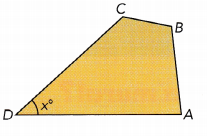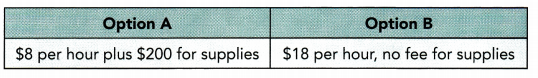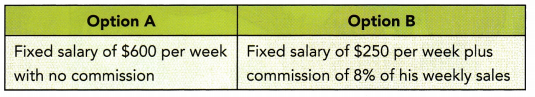# Math in Focus Grade 7 Chapter 4 Review Test Answer Key

Practice the problems of Math in Focus Grade 7 Workbook Answer Key Chapter 4 Review Test to score better marks in the exam.

## Math in Focus Grade 7 Course 2 A Chapter 4 Review Test Answer Key

Concepts and Skills

Solve each equation.

Question 1.
8x – 7 = 17
x = 3

Explanation:
Given, 8x – 7 = 17

8x – 7 + 7 = 17 + 7

8x = 24

Divide 8 on both sides.

8x ÷ 8 = 24 ÷ 8

x = 3

Question 2.
4 – 6x = 8
x = -(2/3)

Explanation:
Given, 4 – 6x = 8

Subtract 4 on both sides.

4 – 6x – 4 = 8 – 4

-6x = 4

Divide -6 on both sides.

-6x ÷ -6 = 4 ÷ -6

x = -(2/3)

Question 3.
6 – $$\frac{y}{3}$$ = 0
y = 18

Explanation:
Give, 6 – (y/3) = 0

Subtract 6 on both sides.

6 – (y/3) – 6= 0 – 6

-(y/3) = -6

(y/3) = 6

Multiply 3 on both sides.

(y/3) × 3 = 6 × 3

y = 18

Question 4.
3 – 3.6x = 4.2
x = -0.333

Explanation:
Given, 3 – 3.6x = 4.2

Subtract 3 on both sides.

3 – 3.6x – 3  = 4.2 – 3

-3.6x = 1.2

Divide  -3.6 on both sides.

-3.6x ÷ -3.6 = 1.2 ÷ -3.6

x = -0.333

Question 5.
7x – 5 = 3x + 4
x = (3/2)

Explanation:
Given, 7x – 5 = 3x + 4

7x – 5 + 5 = 3x + 4 + 5

7x = 3x + 9

Subtract 3x on both sides.

7x – 3x = 3x + 9 – 3x

4x  = 9

Divide 4  on both sides.

4x ÷ 4 = 9 ÷ 4

x = (9/4)

x = (3/2)

Question 6.
$$\frac{7}{10}$$y – $$\frac{1}{5}$$ = $$\frac{3}{5}$$y + $$\frac{6}{5}$$
y = 14

Explanation:
(7/10)y – (1/5) = (3/5)y + (6/5)

Add (1/5) on the both sides.

(7/10)y – (1/5) + (1/5) = (3/5)y + (6/5) + (1/5)

(7/10)y = (3/5)y + (7/5)

Subtract (3/5)y on both sides.

(7/10)y – (3/5)y = (3/5)y +(7/5) – (3/5)y

(7/10)y – ((3×2)/10)y =(7/5)

(7/10)y – (6/10)y =(7/5)

(y/10) = (7/5)

Multiply 1y = 140 on both sides.

(y/10) × 10 = (7/5) × 10

y = 14

Question 7.
3.4y – 5.2 – 3y = 2
y = 18

Explanation:
Given, 3.4y – 5.2 – 3y = 2

0.4y – 5.2 = 2

0.4y – 5.2 + 5.2 = 2 + 5.2

0.4y = 7.2

Divide 0.4 on both sides.

0.4y ÷ 0.4 = 7.2 ÷ 0.4

y = 18

Question 8.
15y – 4(2y – 3) = -2
y = -2

Explanation:
Given, 15y – 4(2y – 3) = -2

15y – 8y + 12 = -2

7y + 12 = -2

Subtract 12 on both sides.

7y + 12 – 12= -2 – 12

7y = -14

Divide 7 on both sides.

7y ÷ 7= -14 ÷ 7

y = -2

Question 9.
$$\frac{1}{4}$$(x + 3) + $$\frac{3}{8}$$x = $$\frac{13}{4}$$
x = 4

Explanation:
Given, (1/4)(x + 3) + (3/8)x = (13/4)

(1/4)x + (3/4) + (3/8)x = (13/4)

Subtract (3/4) on both sides.

(1/4)x + (3/4) + (3/8)x – (3/4) = (13/4) – (3/4)

(1/4)x + (3/8)x =(13-3)/4

(2 + 3)x/8 = 10/4

(5/8)x = (10/4)

Multiply 8 on both sides.

(5/8)x × 8 = (10/4) × 8

5x = 20

Divide 5 on both sides.

5x ÷ 5 = 20 ÷ 5

x = 4

Question 10.
0.4(x + 0.7) = 0.6x – 4.2
x = 22.4

Explanation:
Given, 0.4(x + 0.7) = 0.6x – 4.2

0.4x + 0.28 =0.6x – 4.2

Add 4.2 on the both sides.

0.4x +0.28 + 4.2 =0.6x – 4.2 + 4.2

0.4x + 4.48 = 0.6x

Subtract 0.4x on the both sides.

0.4x + 4.48 – 0.4x = 0.6x – 0.4x

4.48 = 0.2x

0.2x = 4.48

Divide o.2 on both sides.

0.2x ÷ 0.2 = 4.48 ÷ 0.2

x = 22.4

Solve each inequality. Graph each solution set.

Question 11.
4x – 3 > 1
x > 1

Explanation:
Given, 4x – 3 > 1

4x – 3 + 3 > 1 + 3

4x > 4

x > 1

Question 12.
6 ≤ 1 – 5x
-1 ≤ x

Explanation:
Given, 6 ≤ 1 – 5x

Subtract 6 on both sides.

6 – 6 ≤ 1 – 5x – 6

0 ≤ -5x – 5

0 ≤ -5 (x + 1)

Divide -5 on both sides.

0 ÷ -5  ≤ -5 (x + 1) ÷ -5

0 ≤ (x + 1)

-1 ≤ x

Question 13.
$$\frac{2}{3}$$ – $$\frac{x}{6}$$ ≥ –$$\frac{1}{2}$$
x ≤ 1

Explanation:
(2/3) – (x/6) ≥ (1/2)

Subtract (2/3) on both sides.

(2/3) – (x/6) – (2/3) ≥ (1/2) – (2/3)

-(x/6) ≥ (3 – 4/6)

-(x/6) ≥ -(1/6)

Multiply -6 on both sides.

-(x/6) × (-6) ≥ -(1/6) × (-6)

x ≤ 1

Question 14.
-6.9 < 8.1 – 1.5x
x > 10

Explanation:
Given, -6.9 < 8.1 – 1.5x

Subtract 8.1 on both sides.

-6.9 – 8.1 < 8.1 – 1.5x – 8.1

-15 < -1.5x

1.5x > 15

Divide 1.5 on both sides.

1.5x ÷ 1.5 > 15 ÷ 1.5

x > 10

Question 15.
9y – 5 ≤ 4y + 15
y ≤  4

Explanation:
Given, 9y – 5 ≤ 4y + 15

Subtract 4y on both sides.

9y – 5 – 4y ≤ 4y + 15 – 4y

5y – 5 ≤ 15

5y – 5 + 5 ≤ 15 + 5

5y ≤ 20

Divide 5 on both sides.

5y ÷ 5 ≤ 20 ÷ 5

y ≤  4

Question 16.
$$\frac{7}{9}$$x – $$\frac{2}{3}$$ > $$\frac{1}{6}$$x + 3
x > 6

Explanation:
Given, (7/9)x – (2/3) > (1/6)x + 3

Subtract (1/6)x on both sides.

(7/9)x – (2/3) – (1/6)x > (1/6)x + 3 – (1/6)x

(14 – 3)/18x – (2/3) > 3

11/18x – (2/3) > 3

11/18x- (2/3) + (2/3) > 3 + (2/3)

11/18x > (11/3)

Divide 11 on both sides.

11/18x ÷ 11 > (11/3) ÷ 11

(1/18)x > (1/3)

Multiply 3 on both sides.

(1/18)x × 3  > (1/3) × 3

(1/6)x > 1

Multiply 6 on both sides.

(1/6)x × 6 > 1 × 6

x > 6

Question 17.
12.9 < 0.3(5.3 – x)

Question 18.
3(x + 1) > 5x + 7

Question 19.
$$\frac{1}{5}$$(4x – 1) ≥ $$\frac{2}{3}$$x + $$\frac{3}{5}$$
x ≥ 6

Explanation:
Given, (1/5)(4x – 1) ≥ (2/3)x + (3/5)

Multiply 5 on both sides

(1/5)(4x – 1) × 5  ≥ ((2/3)x + (3/5)) × 5

(4x – 1) ≥  (10/3)x + 3

(4x – 1) + 1 ≥  (10/3)x + 3 + 1

4x ≥  (10/3)x + 4

Subtract (10/3)x on both sides.

4x – (10/3)x ≥  (10/3)x + 4 – (10/3)x

(12 – 10)x/3 ≥ 4

(2/3)x ≥ 4

Multiply 3 on both sides.

(2/3)x × 3 ≥ 4 × 3

2x ≥ 12

Divide 2 on both sides.

2x ÷ 2  ≥ 12 ÷ 2

x ≥ 6

Question 20.
4(3 – 0.1x) ≤ 15 – 0.6x
x ≤ 15

Explanation:
Given, 4(3 – 0.1x) ≤ 15 – 0.6x

12 -0.4x ≤ 15 – 0.6x

Subtract 12 on both sides.

12 -0.4x – 12 ≤ 15 – 0.6x – 12

-0.4x ≤ 3 -0.6x

-0.4x + 0.6x ≤ 3 -0.6x + 0.6x

0.2x ≤ 3

Divide 0.2 on both sides.

0.2x ÷ 0.2 ≤ 3 ÷ 0.2

x ≤ 15

Problem Solving

Write an equation for questions 21 to 25. Solve and show your work.

Question 21.
Aiden wrote a riddle: Five less than $$\frac{1}{5}$$ times a number is the same as the sum of the number and $$\frac{1}{3}$$. Find the number.
x = -(20/3)

Explanation:
Let us consider the number be ‘x’.

(1/5)x – 5 = x + (1/3)

(1/5)x – 5 + 5 = x + (1/3) + 5

(1/5)x = x + (16/3)

Subtract (1/5)x on both sides.

(1/5)x – (1/5)x  = x + (16/3) – (1/5)x

0 = (4/5)x + (16/3)

(4/5)x = (- 16/3)

Multiply 5 on both sides.

(4/5)x × 5 = (- 16/3) × 5

4x = – 80/3

Divide 4 on both sides.

4x ÷ 4 = (- 80/3) ÷ 4

x = -(20/3)

Question 22.
Mary is 6 years older than her sister Kelly. The sum of their ages is 48. How old is Kelly?
Kelly’s age, x = 21

Explanation:
Let us consider the age of the Kelly be ‘x’.

Age of Mary is  x +6

Sum of their ages = 48

x +x + 6 = 48

2x + 6 = 48

Subtract 6 on both sides.

2x + 6 – 6 = 48 – 6

2x = 42

Divide 2 on  both sides.

2x ÷ 2 = 42 ÷ 2

x = 21

Question 23.
The sum of the page numbers of two facing pages in a book is 145. What are the page numbers?
x = 72; x + 1 = 73.

72, 73 are the page numbers.

Explanation:
Let us consider the numbers be x and x+1.

x + x +1 = 145

2x + 1 = 145

Subtract 1 on both sides.

2x + 1 – 1 = 145 – 1

2x = 144

Divide 2 on both sides.

2x ÷ 2 = 144 ÷ 2

x = 72

x + 1 = 73

The sum of numbers of two facing pages in a book = 145

Question 24.
The perimeter of an equilateral triangle is 6$$\frac{3}{4}$$ inches. Find the length of each side of the equilateral triangle.
Length of each side, a = (3/2)

Explanation:
Given, The perimeter of an equilateral triangle is 6(3/4).

3a = 6(3/4)

Divide 3 on both sides.

3a ÷ 3 = 6(3/4) ÷ 3

a = (6/4)

a = (3/2)

Question 25.
The sum of the interior angle measures of a quadrilateral is 360°. The measure of angle A is three times the measure of angle D. The measure of angle 6 is four times that of angle D. The measure of angle C is 24° more than angle B. Find the measure of each angle of the quadrilateral.Write an inequality for each question. Solve and show your work.

Question 26.
Laura wants the average amount of money she spends each day on her four-day vacation to be no more than $64. On the first three days, she spends$71, $62, and$59. What is the greatest amount of money she can spend on the fourth day?
64

Explanation:
Given, Mean = ax=n(Mean)(a1+...+an)

= 4(64) – (71 + 62 + 59)

= 256 – 192

= 64

Question 27.
Kevin plans to sign up for p hours of training at a culinary school. The school offers two payment options as shown below.For how many hours of training is Option B less expensive than Option A?
x < 20, 20 hours.

Explanation:
Let us consider number of hours be ‘x’.

18x + 0 < 8x +200

18x < 8x + 200

Subtract 8x on both sides.

18x – 8x < 8x + 200 – 8x

10x < 200

Divide 10 on both sides.

10x ÷ 10 < 200 ÷ 10

x < 20

Question 28.
Peter has found a job in a computer store. As shown below, he has two options for how he will be paid. The commission he makes for Option B is based on his weekly sales. For example, if his sales total $1,000 a week, he receives his base salary of$250 plus 8% of $1,000.Peter is thinking about Option B. What would his weekly sales need to be for him to make at least as much as he would for Option A? Answer: The sales should be nearly above 4000$ per week to get more than Option A. Option A is better than Option B.

Explanation:

Let us consider ‘x’ be the number of sales.

600 ≥ 250 + 0.08x

Subtract 250 on both sides.

600 – 250 ≥ 250 + (0.08×1000)x – 250

350 ≥ 80x

Divide 80 on both sides.

350 ÷ 80 ≥ 80x ÷ 80

4 ≥ x

The sales should be nearly above 4000$per week to get more than Option A. so Option A is better than Option B. Question 29. The school events committee is planning to buy a banner and some helium balloons for graduation night. A store charges them$35 for the banner and $3.50 for each helium balloon. If the committee has at most$125 to spend, how many helium balloons can they buy?
x ≤ 25

Explanation:
Let us consider number of helium balloons be ‘x’.

35 + 3.5x ≤ 125

Subtract 35 on both sides.

35 + 3.5x – 35 ≤ 125 – 35

3.5x ÷ 3.5  ≤ 90 ÷ 3.5

Divide 3.5 on both sides.

3.5x ≤ 90

x ≤ 25

Question 30.
The coach of the field hockey team can spend at most $475 on new team uniforms. The coach will order the uniforms online and pay a mailing cost of$6.50. If each uniform costs \$29, how many uniforms can the coach order?
x ≤ 16, 16 uniforms.

Explanation:
Let us consider the number of uniforms be ‘x’.

6.5 + 29x ≤ 475

Subtract 6.5 on both sides.

6.5 + 29x – 6.5 ≤ 475 – 6.5

29x ≤ 468.5

Divide 29 on both sides.

29x ÷ 29 ≤ 468.5 ÷ 29

x ≤ 16